PREDPPLOT Statement
PREDPPLOT <VAR= variable> <options> ;

The PREDPPLOT statement plots the predicted probability against a single continuous variable (dose variable) in the MODEL statement for both the binomial model and the multinomial model. Confidence limits are available only for the binomial model. An attached box on the right side of the plot is used to label predicted probability curves with the names of their levels for the multinomial model. You can specify the color of this box by using the CLABBOX= option.

VAR=variable

specifies a single continuous variable (dose variable) in the independent variable list of the MODEL statement. If a VAR= variable is not specified, the first single continuous variable in the independent variable list of the MODEL statement is used. If such a variable does not exist in the independent variable list of the MODEL statement, an error is reported.

The predicted probability is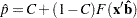for the binomial model and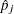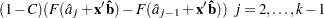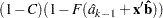for the multinomial model withresponse levels, whereis the cumulative distribution function used to model the probability,is the vector of the covariates,are the estimated ordinal intercepts with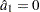,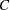is the threshold parameter, either known or estimated from the model, and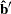is the vector of estimated parameters.

To plot
(or) as a function of a continuous variable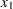, the remaining covariatesmust be specified. You can use the XDATA= option to provide the values of(see the XDATA= option in the PROC PROBIT statement for details), or use the default values that follow these rules:

• If the effect contains a continuous variable (or variables), the overall mean of this effect is used.

• If the effect is a single classification variable, the highest level of the variable is used.

options

enable you to plot the observed data and add features to the plot.

You can use options in the PREDPPLOT statement to do the following:

• superimpose specification limits

• suppress or add observed data points for the binomial model

• suppress or add confidence limits for the binomial model

• specify the levels for which predicted probability curves are requested for the multinomial model

• specify graphical enhancements (such as color or text height)

### Summary of Options

Table 74.26 through Table 74.32 list all options by function. The Dictionary of Options describes each option in detail.

#### PREDPPLOT Options

 LEVEL=(character-list) Specifies the names of the levels for which the predicted probability curves are requested (only for the multinomial model) NOCONF Suppresses confidence limits NODATA Suppresses observed data points on the plot NOTHRESH Suppresses the threshold line THRESHLABPOS=value Specifies the position for the label of the threshold line

#### General Options

 CAXIS=color Specifies color for the axes CFIT=color Specifies color for fitted curves CFRAME=color Specifies color for frame CGRID=color Specifies color for grid lines CHREF=color Specifies color for HREF= lines CLABBOX=color Specifies color for label box CTEXT=color Specifies color for text CVREF=color Specifies color for VREF= lines
 ANNOTATE=SAS-data-set Specifies an Annotate data set INBORDER Requests a border around plot LFIT=linetype Specifies line style for fitted curves and confidence limits LGRID=linetype Specifies line style for grid lines NOFRAME Suppresses the frame around plotting areas NOGRID Suppresses grid lines NOFIT Suppresses fitted curves NOHLABEL Suppresses horizontal labels NOHTICK Suppresses horizontal ticks NOVTICK Suppresses vertical ticks TURNVLABELS Vertically strings out characters in vertical labels WFIT=n Specifies thickness for fitted curves WGRID=n Specifies thickness for grids WREFL=n Specifies thickness for reference lines
 HAXIS=value1 to value2 Specifies tick mark values for horizontal axis HOFFSET=value Specifies offset for horizontal axis HLOWER=value Specifies lower limit on horizontal axis scale HUPPER=value Specifies upper limit on horizontal axis scale NHTICK=n Specifies number of ticks for horizontal axis NVTICK=n Specifies number of ticks for vertical axis VAXIS=value1 to value2 Specifies tick mark values for vertical axis VAXISLABEL=’label’ Specifies label for vertical axis VOFFSET=value Specifies offset for vertical axis VLOWER=value Specifies lower limit on vertical axis scale VUPPER=value Specifies upper limit on vertical axis scale WAXIS=n Specifies thickness for axis
 DESCRIPTION=’string’ Specifies description for graphics catalog member NAME=’string’ Specifies name for plot in graphics catalog
 FONT=font Specifies software font for text HEIGHT=value Specifies height of text used outside framed areas INFONT=font Specifies software font for text inside framed areas INHEIGHT=value Specifies height of text inside framed areas
 HREF<(INTERSECT)>=value-list Requests horizontal reference line HREFLABELS=(’label1’,...,’labeln’) Specifies labels for HREF= lines HREFLABPOS=n Specifies vertical position of labels for HREF= lines LHREF=linetype Specifies line style for HREF= lines LVREF=linetype Specifies line style for VREF= lines VREF<(INTERSECT)>=value-list Requests vertical reference line VREFLABELS=(’label1’,...,’labeln’) Specifies labels for VREF= lines VREFLABPOS=Specifies horizontal position of labels for VREF= lines

### Dictionary of Options

The following entries provide detailed descriptions of the options in the PREDPPLOT statement.

ANNOTATE=SAS-data-set
ANNO=SAS-data-set

specifies an Annotate data set, as described in SAS/GRAPH Software: Reference, that enables you to add features to the predicted probability plot. The ANNOTATE= data set you specify in the PREDPPLOT statement is used for all plots created by the statement.

CAXIS=color
CAXES=color

specifies the color used for the axes and tick marks. This option overrides any COLOR= specifications in an AXIS statement. The default is the first color in the device color list.

CFIT=color

specifies the color for the fitted predicted probability curves. The default is the first color in the device color list.

CFRAME=color
CFR=color

specifies the color for the area enclosed by the axes and frame. This area is not shaded by default.

CGRID=color

specifies the color for grid lines. The default is the first color in the device color list.

CHREF=color
CH=color

specifies the color for lines requested by the HREF= option. The default is the first color in the device color list.

CTEXT=color

specifies the color for tick mark values and axis labels. The default is the color specified for the CTEXT= option in the most recent GOPTIONS statement.

CVREF=color
CV=color

specifies the color for lines requested by the VREF= option. The default is the first color in the device color list.

DESCRIPTION=’string’
DES=’string’

specifies a description, up to 40 characters, that appears in the PROC GREPLAY master menu. The default is the variable name.

FONT=font

specifies a software font for reference line and axis labels. You can also specify fonts for axis labels in an AXIS statement. The FONT= font takes precedence over the FTEXT= font specified in the most recent GOPTIONS statement. Hardware characters are used by default.

HAXIS=value1 to value2 <by value3>

specifies tick mark values for the horizontal axis; value1, value2, and value3 must be numeric, and value1 must be less than value2. The lower tick mark is value1. Tick marks are drawn at increments of value3. The last tick mark is the greatest value that does not exceed value2. If value3 is omitted, a value of 1 is used.

Examples of HAXIS= lists follow:

```   haxis = 0 to 10
haxis = 2 to 10 by 2
haxis = 0 to 200 by 10
```
HEIGHT=value

specifies the height of text used outside framed areas.

HLOWER=value

specifies the lower limit on the horizontal axis scale. The HLOWER= option specifies value as the lower horizontal axis tick mark. The tick mark interval and the upper axis limit are determined automatically. This option has no effect if the HAXIS= option is used.

HOFFSET=value

specifies the offset for the horizontal axis. The default value is 1.

HUPPER=value

specifies value as the upper horizontal axis tick mark. The tick mark interval and the lower axis limit are determined automatically. This option has no effect if the HAXIS= option is used.

HREF <(INTERSECT)> =value-list

requests reference lines perpendicular to the horizontal axis. If (INTERSECT) is specified, a second reference line perpendicular to the vertical axis is drawn that intersects the fit line at the same point as the horizontal axis reference line. If a horizontal axis reference line label is specified, the intersecting vertical axis reference line is labeled with the vertical axis value. See also the CHREF=, HREFLABELS=, and LHREF= options.

HREFLABELS=’label1’,...,’labeln’
HREFLABEL=’label1’,...,’labeln’
HREFLAB=’label1’,...,’labeln’

specifies labels for the lines requested by the HREF= option. The number of labels must equal the number of lines. Enclose each label in quotes. Labels can be up to 16 characters.

HREFLABPOS=n

specifies the vertical position of labels for HREF= lines. The following table shows valid values for n and the corresponding label placements.

 n label placement 1 Top 2 Staggered from top 3 Bottom 4 Staggered from bottom 5 Alternating from top 6 Alternating from bottom
INBORDER

requests a border around predicted probability plots.

LEVEL=(character-list)
ORDINAL= (character-list)

specifies the names of the levels for which predicted probability curves are requested. Names should be quoted and separated by space. If there is no correct name provided, no fitted probability curve is plotted.

LFIT=linetype

specifies a line style for fitted curves and confidence limits. By default, fitted curves are drawn by connecting solid lines (linetype = 1) and confidence limits are drawn by connecting dashed lines (linetype = 3).

LGRID=linetype

specifies a line style for all grid lines. The value for linetype is between 1 and 46. The default is 35.

LHREF=linetype
LH=linetype

specifies the line type for lines requested by the HREF= option. The default is 2, which produces a dashed line.

LVREF=linetype
LV=linetype

specifies the line type for lines requested by the VREF= option. The default is 2, which produces a dashed line.

NAME=’string’

specifies a name for the plot, up to eight characters, that appears in the PROC GREPLAY master menu. The default is ’PROBIT’.

NOCONF

suppresses confidence limits from the plot. This works only for the binomial model. Confidence limits are not plotted for the multinomial model.

NODATA

suppresses observed data points from the plot. This works only for the binomial model. The data points are not plotted for the multinomial model.

NOFIT

suppresses the fitted predicted probability curves.

NOFRAME

suppresses the frame around plotting areas.

NOGRID

suppresses grid lines.

NOHLABEL

suppresses horizontal labels.

NOHTICK

suppresses horizontal tick marks.

NOTHRESH

suppresses the threshold line.

NOVLABEL

suppresses vertical labels.

NOVTICK

suppresses vertical tick marks.

THRESHLABPOS=n

specifies the horizontal position of labels for the threshold line. The following table shows valid values for n and the corresponding label placements.

 n label placement 1 Left 2 Right
VAXIS=value1 to value2 <by value3>

specifies tick mark values for the vertical axis; value1, value2, and value3 must be numeric, and value1 must be less than value2. The lower tick mark is value1. Tick marks are drawn at increments of value3. The last tick mark is the greatest value that does not exceed value2. This method of specification of tick marks is not valid for logarithmic axes. If value3 is omitted, a value of 1 is used.

Examples of VAXIS= lists follow:

```   vaxis = 0 to 10
vaxis = 0 to 2 by .1
```
VAXISLABEL=’string’

specifies a label for the vertical axis.

VLOWER=value

specifies the lower limit on the vertical axis scale. The VLOWER= option specifies value as the lower vertical axis tick mark. The tick mark interval and the upper axis limit are determined automatically. This option has no effect if the VAXIS= option is used.

VREF=value-list

requests reference lines perpendicular to the vertical axis. If (INTERSECT) is specified, a second reference line perpendicular to the horizontal axis is drawn that intersects the fit line at the same point as the vertical axis reference line. If a vertical axis reference line label is specified, the intersecting horizontal axis reference line is labeled with the horizontal axis value. See also the CVREF=, LVREF=, and VREFLABELS= options.

VREFLABELS=’label1’,...,’labeln’
VREFLABEL=’label1’,...,’labeln’
VREFLAB=’label1’,...,’labeln’

specifies labels for the lines requested by the VREF= option. The number of labels must equal the number of lines. Enclose each label in quotes. Labels can be up to 16 characters.

VREFLABPOS=n

specifies the horizontal position of labels for VREF= lines. The following table shows valid values for n and the corresponding label placements.

 n label placement 1 Left 2 Right
VUPPER=value

specifies the upper limit on the vertical axis scale. The VUPPER= option specifies value as the upper vertical axis tick mark. The tick mark interval and the lower axis limit are determined automatically. This option has no effect if the VAXIS= option is used.

WAXIS=n

specifies line thickness for axes and frame. The default value is 1.

WFIT=n

specifies line thickness for fitted curves. The default value is 1.

WGRID=n

specifies line thickness for grids. The default value is 1.

WREFL=n

specifies line thickness for reference lines. The default value is 1.